﻿ 人像抠图无监督语义精修算法

# 人像抠图无监督语义精修算法Unsupervised Semantic Human Matting Refinement

Abstract: Aiming at the problems of human matting algorithm, which does not use trimap as a prior knowledge, such as redundant interference information, rough portrait edge contour, and easy confusion between objects carried by human body and the background, an unsupervised semantic matting algorithm for human matting is proposed. The algorithm is composed of human border sensing module and unsupervised semantic refinement module. The portraits border sensing module firstly uses the pedestrian detection model to identify all the portraits, and combines the border sensing algorithm to remove the redundant interference information. Unsupervised semantic refinement module uses unsupervised semantic segmentation model to extract features, and then uses semantic refinement algorithm to repair the portrait contour. Experiments show that in the self-made longterm portrait data set, the mainstream portrait matting algorithm is used as the baseline, and the unsupervised semantic refinement algorithm for portrait matting is added. The effect is significantly improved, and the objects carried by the human body can also be accurately identified. The outline is also clearer. At the same time, in the portrait data set, the effect is also improved to some extent, indicating that the algorithm is also generalized.

1. 引言

${I}_{i}={\alpha }_{i}{F}_{i}+\left(1-{\alpha }_{i}\right){B}_{i},\text{}{\alpha }_{i}\in \left[0,1\right]$ (1)

2. 人像抠图无监督语义精修算法

2.1. 人像边框感知模块

Input: $B=\left({x}_{1}\text{,}{x}_{2},\text{}{y}_{1},\text{}{y}_{2}\right)$ 表示人像边框，mask表示透明度遮罩。

Output: B'表示修正后的人像边框。

1) initialize set ${B}^{\prime }=0$

2) for $i←{x}_{1}$ to x2 do

3) while $mas{k}_{i,{y}_{1}}>0$

4) do ${y}_{1}←{y}_{1}+1$ end

5) while $mas{k}_{i,{y}_{\text{2}}}>0$

6) do ${y}_{\text{2}}←{y}_{\text{2}}-1$ end

7) end for

8) for $i←{y}_{2}$ to y1 do

9) while $mas{k}_{{x}_{1},i}>0$

10) do ${x}_{1}←{x}_{1}-1$ end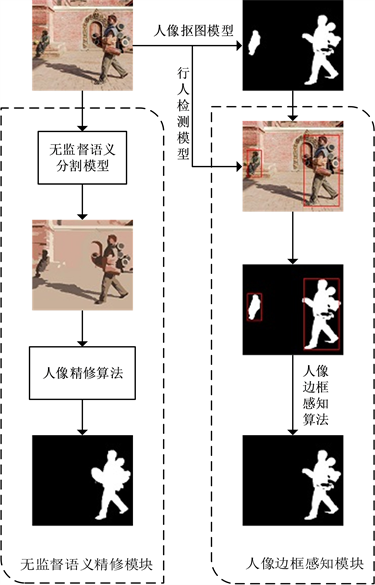Figure 1. The flow chart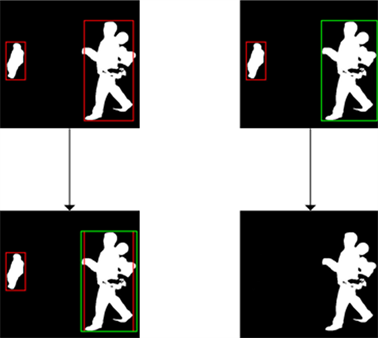(a) 人像边框内修正算法 (b) 人像边框外修正算法

Figure 2. Portrait border sensing algorithm flow chart

11) while $mas{k}_{{x}_{2},i}>0$

12)do ${x}_{2}←{x}_{2}+1$ end

13) end for

14) ${B}^{\prime }←\left({x}_{1}，\text{}{x}_{2}，\text{}{y}_{1}，\text{}{y}_{2}\right)$

2.2. 无监督语义精修模块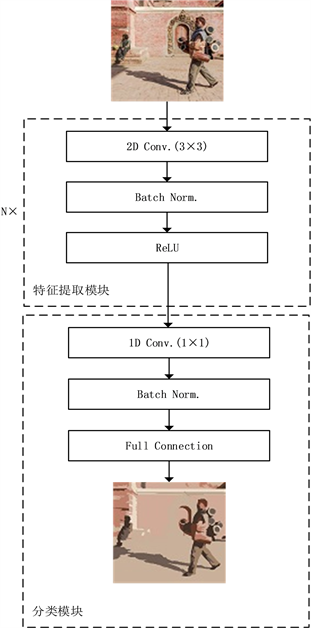Figure 3. Unsupervised portrait semantic segmentation network

${h}_{i}=\mathrm{Re}\text{LU}\left(\text{BN}\left(con{v}_{3×3}\left({h}_{i-1}\right)\right)\right)$ (2)

$G=\text{classification}\left(\text{BN}\left(con{v}_{1×1}\left({h}_{M}\right)\right)\right)$ (3)

$\text{Loss}=\frac{1}{N}\underset{i=1}{\overset{N}{\sum }}\left(-\underset{j=1}{\overset{k}{\sum }}{y}_{i,j}\mathrm{log}\frac{{\text{e}}^{{G}_{i,j}}}{{\sum }_{l=1}^{k}{\text{e}}^{{G}_{i,l}}}\right)$ (4)

RGB三通道图像经过无监督人像语义分割网络后每个像素点被分为q类。相同类别且连续的像素点构成区域Pk，其中 $k\in \left\{1,2,\cdots ,q\right\}$。人像精修算法的核心在于统计mask在每个区域Pk中前景与背景的个数，本文把像素点为黑色设为背景，像素点为白色设为前景。如果mask在区域Pk中前景的数量与背景的数量的比值大于θ，则把mask在区域Pk中的值都设置为前景的值，如果前景的数量与背景的数量的比值小于 $\left(\text{1}-\theta \right)$，则把mask在区域Pk中的值都设置为背景的值。其中θ为超参数。该算法可以修改mask中的属于背景信息的前景信息，同时可以细化人像及携带物品的边缘轮廓。

3. 实验设计及结果分析

3.1. 实验准备

3.2. 实验结果与分析

$\text{MSE}=\frac{\text{1}}{n}\underset{i=1}{\overset{n}{\sum }}{\left({x}_{i}-{y}_{i}\right)}^{2}$ (5)

$\text{SAD}=\frac{\text{1}}{n}\underset{i=1}{\overset{n}{\sum }}|{x}_{i}-{y}_{i}|$ (6)Table 1. The results of two pre-classification algorithms in different hyperparameters θTable 2. Comparison of experimental results in the perspective portrait datasetTable 3. Results of two module ablation experiments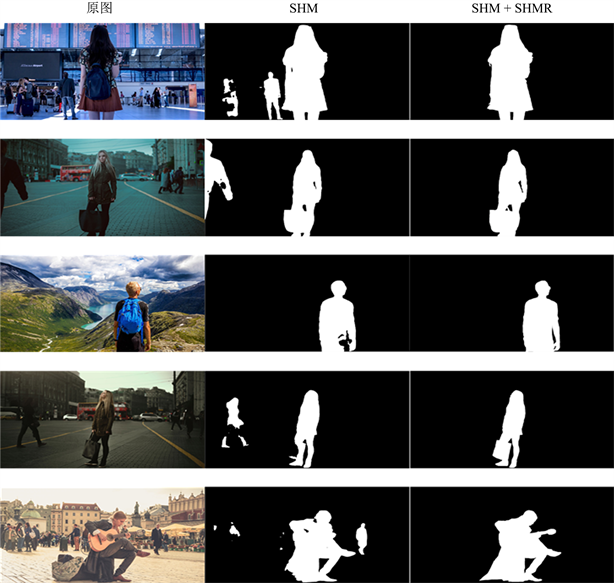Figure 4. Experimental renderings in the perspective portrait datasetTable 4. Comparison of experimental results in the data set of half-length portraits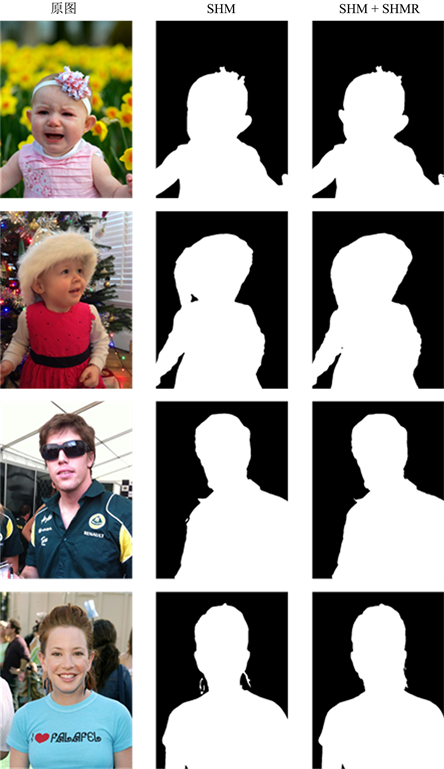Figure 5. Experimental renderings of the bust portrait data set

4. 结束语

 Wu, X., Fang, X.N., Chen, T., et al. (2020) JMNet: A Joint Matting Network for Automatic Human Matting. Computa-tion Visual Media, 6, 215-224.
https://doi.org/10.1007/s41095-020-0168-6

 Badrinarayanan, V., Kendall, A. and Cipolla, R. (2019) SegNet: A Deep Convolutional Encoder-Decoder Architecture for Image Segmentation. IEEE Transactions on Pattern Analysis and Machine Intelligence, 39, 2481-2495.
https://doi.org/10.1109/TPAMI.2016.2644615

 Long, J., Shelhamer, E. and Darrell, T. (2015) Fully Convolu-tional Networks for Semantic Segmentation. Proceedings of 2015 IEEE Conference on Computer Vision and Pattern Recognition, Boston, 7-12 June 2015, 3431-3440.
https://doi.org/10.1109/CVPR.2015.7298965

 Aksoy, Y., Aydın, T.O. and Pollefeys, M. (2017) Designing Effective Inter-Pixel Information Flow for Natural Image Matting. Proceedings of 2017 IEEE Conference on Computer Vision and Pattern Recognition, Honolulu, 21-26 July 2017, 29-37.
https://doi.org/10.1109/CVPR.2017.32

 Chen, Q.F., et al. (2013) KNN Matting. IEEE Transactions on Pattern Analysis & Machine Intelligence, 35, 2175-2188.
https://doi.org/10.1109/TPAMI.2013.18

 Cai, S., Zhang, X., Fan, H., et al. (2019) Disentangled Image Matting. The IEEE International Conference on Computer Vision, Seoul, 27-28 October 2019, 8818-8827.
https://doi.org/10.1109/ICCV.2019.00891

 Shen, X., Tao, X., Gao, H., et al. (2016) Deep Automatic Portrait Matting. European Conference on Computer Vision, Amsterdam, 8-16 October 2016, 92-107.
https://doi.org/10.1007/978-3-319-46448-0_6

 Cho, D., Tai, Y.W. and Kweon, I. (2016) Natural Im-age Matting Using Deep Convolutional Neural Networks. The European Conference on Computer Vision, Amsterdam, 8-16 October 2016, 626-643.
https://doi.org/10.1007/978-3-319-46475-6_39

 Levin, A. (2006) A Closed Form Solution to Natural Image Matting. Proceedings of 2006 IEEE Conference on Computer Vision and Pattern Recognition, New York, 17-22 June 2006, 228-242.
https://doi.org/10.1109/TPAMI.2007.1177

 Ning, X., Price, B., Cohen, S. and Huang, T. (2017) Deep Image Matting. Proceedings of 2017 IEEE Conference on Computer Vision and Pattern Recognition, Honolulu, 21-26 July 2017, 2970-2979.

 Lutz, S., Amplianitis, K. and Smolic, A. (2018) AlphaGAN: Generative Adversarial Networks for Natural Image Matting. British Machine Vision Conference, Newcastle, 3-6 September 2018, 259.

 Chen, Q., Ge, T., Xu, Y., et al. (2018) Semantic Human Matting. 2018 ACM Multimedia Conference, Seoul, 22-26 October 2018, 618-626.
https://doi.org/10.1145/3240508.3240610

 Liu, J., Yao, Y., Hou, W., et al. (2020) Boosting Semantic Human Matting with Coarse Annotations. Proceedings of 2020 IEEE Conference on Computer Vision and Pattern Recognition, Seattle, 14-19 June 2020, 8560-8569.
https://doi.org/10.1109/CVPR42600.2020.00859

 Redmon, J. and Farhadi, A. (2018) YOLOv3: An Incre-mental Improvement.

 Kanezaki, A. (2018) Unsupervised Image Segmentation by Backpropagation. IEEE Interna-tional Conference on Acoustics, Calgary, 15-20 April 2018, 1543-1547.
https://doi.org/10.1109/ICASSP.2018.8462533

 Kingma, D.P. and Jimmy, B. (2014) Adam: A Method for Stochastic Optimization.

 Felzenszwalb, P.F. and Huttenlocher, D.P. (2004) Efficient Graph-Based Image Segmen-tation. International Journal of Computer Vision, 59, 167-181.
https://doi.org/10.1023/B:VISI.0000022288.19776.77

 Achanta, R., Shaji, A., Smith, K., et al. (2012) SLIC Superpixels Compared to State-of-the-Art Superpixel Methods. IEEE Transactions on Pattern Analysis & Machine Intel-ligence, 34, 2274-2282.
https://doi.org/10.1109/TPAMI.2012.120

Top